The distribution of a (test) statistic T(yrep), or a pair of (test) statistics, over the simulated datasets in yrep, compared to the observed value T(y) computed from the data y. See the Plot Descriptions and Details sections, below, as well as Gabry et al. (2019).

ppc_stat(
y,
yrep,
stat = "mean",
...,
binwidth = NULL,
breaks = NULL,
freq = TRUE
)

ppc_stat_grouped(
y,
yrep,
group,
stat = "mean",
...,
facet_args = list(),
binwidth = NULL,
breaks = NULL,
freq = TRUE
)

ppc_stat_freqpoly(
y,
yrep,
stat = "mean",
...,
facet_args = list(),
binwidth = NULL,
freq = TRUE
)

ppc_stat_freqpoly_grouped(
y,
yrep,
group,
stat = "mean",
...,
facet_args = list(),
binwidth = NULL,
freq = TRUE
)

ppc_stat_2d(y, yrep, stat = c("mean", "sd"), ..., size = 2.5, alpha = 0.7)

ppc_stat_data(y, yrep, group = NULL, stat)

## Arguments

y

A vector of observations. See Details.

yrep

An S by N matrix of draws from the posterior (or prior) predictive distribution. The number of rows, S, is the size of the posterior (or prior) sample used to generate yrep. The number of columns, N is the number of predicted observations (length(y)). The columns of yrep should be in the same order as the data points in y for the plots to make sense. See the Details and Plot Descriptions sections for additional advice specific to particular plots.

stat

A single function or a string naming a function, except for the 2D plot which requires a vector of exactly two names or functions. In all cases the function(s) should take a vector input and return a scalar statistic. If specified as a string (or strings) then the legend will display the function name(s). If specified as a function (or functions) then generic naming is used in the legend.

...

Currently unused.

binwidth

Passed to ggplot2::geom_histogram() to override the default binwidth.

breaks

Passed to ggplot2::geom_histogram() as an alternative to binwidth.

freq

For histograms, freq=TRUE (the default) puts count on the y-axis. Setting freq=FALSE puts density on the y-axis. (For many plots the y-axis text is off by default. To view the count or density labels on the y-axis see the yaxis_text() convenience function.)

group

A grouping variable of the same length as y. Will be coerced to factor if not already a factor. Each value in group is interpreted as the group level pertaining to the corresponding observation.

facet_args

A named list of arguments (other than facets) passed to ggplot2::facet_wrap() or ggplot2::facet_grid() to control faceting. Note: if scales is not included in facet_args then bayesplot may use scales="free" as the default (depending on the plot) instead of the ggplot2 default of scales="fixed".

size, alpha

For the 2D plot only, arguments passed to ggplot2::geom_point() to control the appearance of scatterplot points.

## Value

The plotting functions return a ggplot object that can be further customized using the ggplot2 package. The functions with suffix _data() return the data that would have been drawn by the plotting function.

## Details

For Binomial data, the plots may be more useful if the input contains the "success" proportions (not discrete "success" or "failure" counts).

## Plot Descriptions

ppc_stat(), ppc_stat_freqpoly()

A histogram or frequency polygon of the distribution of a statistic computed by applying stat to each dataset (row) in yrep. The value of the statistic in the observed data, stat(y), is overlaid as a vertical line. More details and example usage of ppc_stat() can be found in Gabry et al. (2019).

ppc_stat_grouped(),ppc_stat_freqpoly_grouped()

The same as ppc_stat() and ppc_stat_freqpoly(), but a separate plot is generated for each level of a grouping variable. More details and example usage of ppc_stat_grouped() can be found in Gabry et al. (2019).

ppc_stat_2d()

A scatterplot showing the joint distribution of two statistics computed over the datasets (rows) in yrep. The value of the statistics in the observed data is overlaid as large point.

Other PPCs: PPC-censoring, PPC-discrete, PPC-distributions, PPC-errors, PPC-intervals, PPC-loo, PPC-overview, PPC-scatterplots

## Examples

y <- example_y_data()
yrep <- example_yrep_draws()
ppc_stat(y, yrep)
#> stat_bin() using bins = 30. Pick better value with binwidth.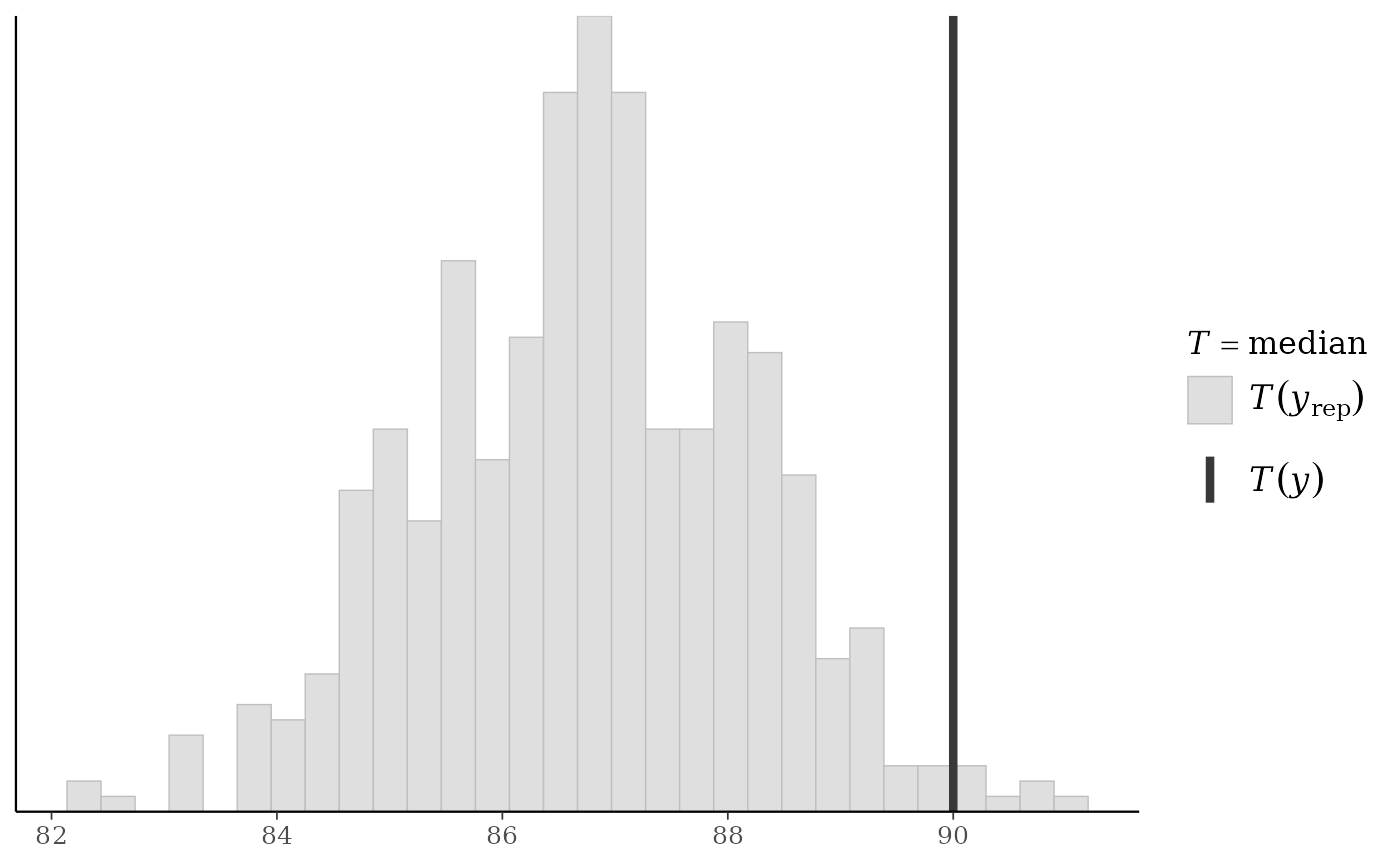ppc_stat(y, yrep, stat = "sd") + legend_none()
#> stat_bin() using bins = 30. Pick better value with binwidth.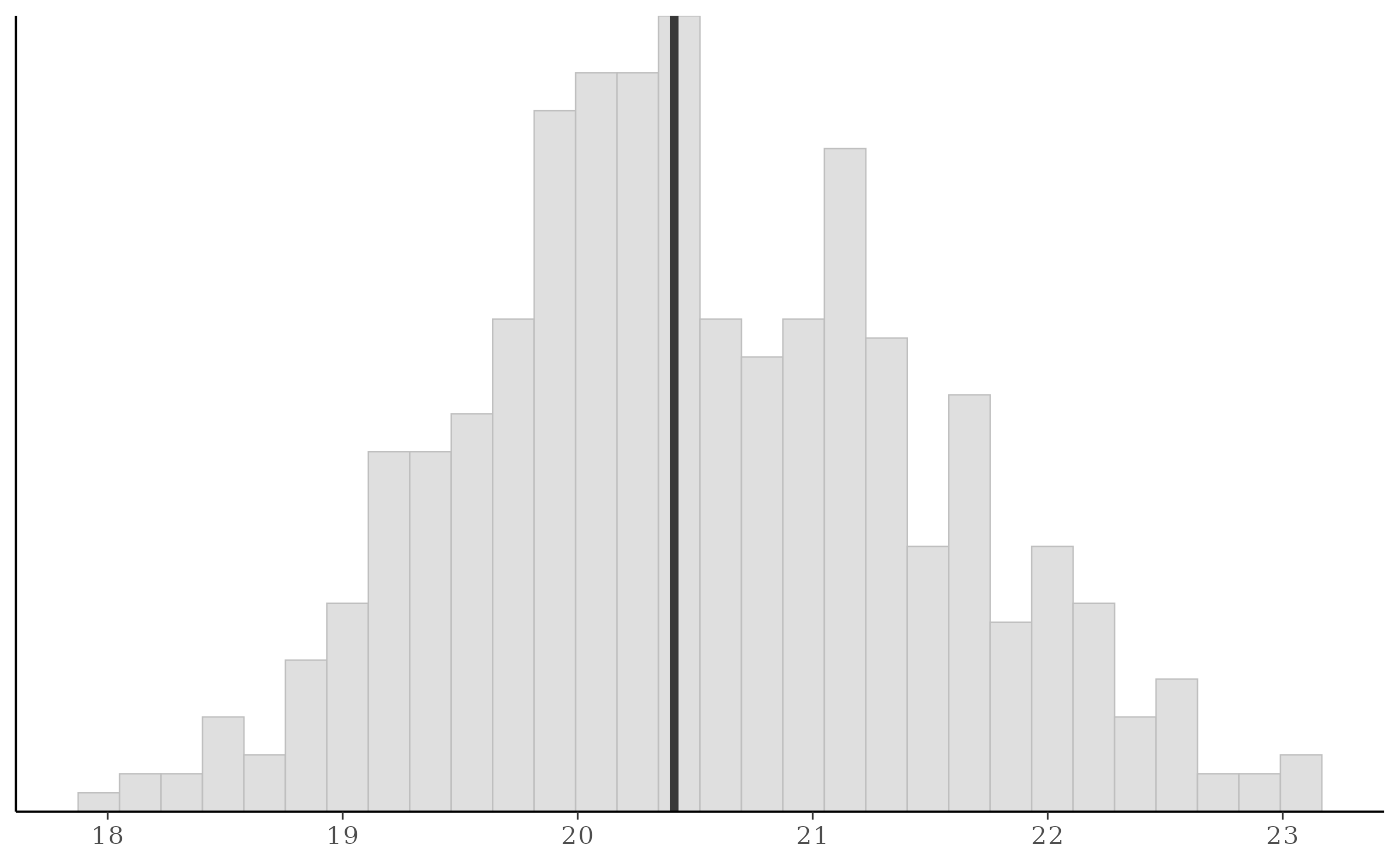# use your own function for the 'stat' argument
color_scheme_set("brightblue")
q25 <- function(y) quantile(y, 0.25)
ppc_stat(y, yrep, stat = "q25") # legend includes function name
#> stat_bin() using bins = 30. Pick better value with binwidth.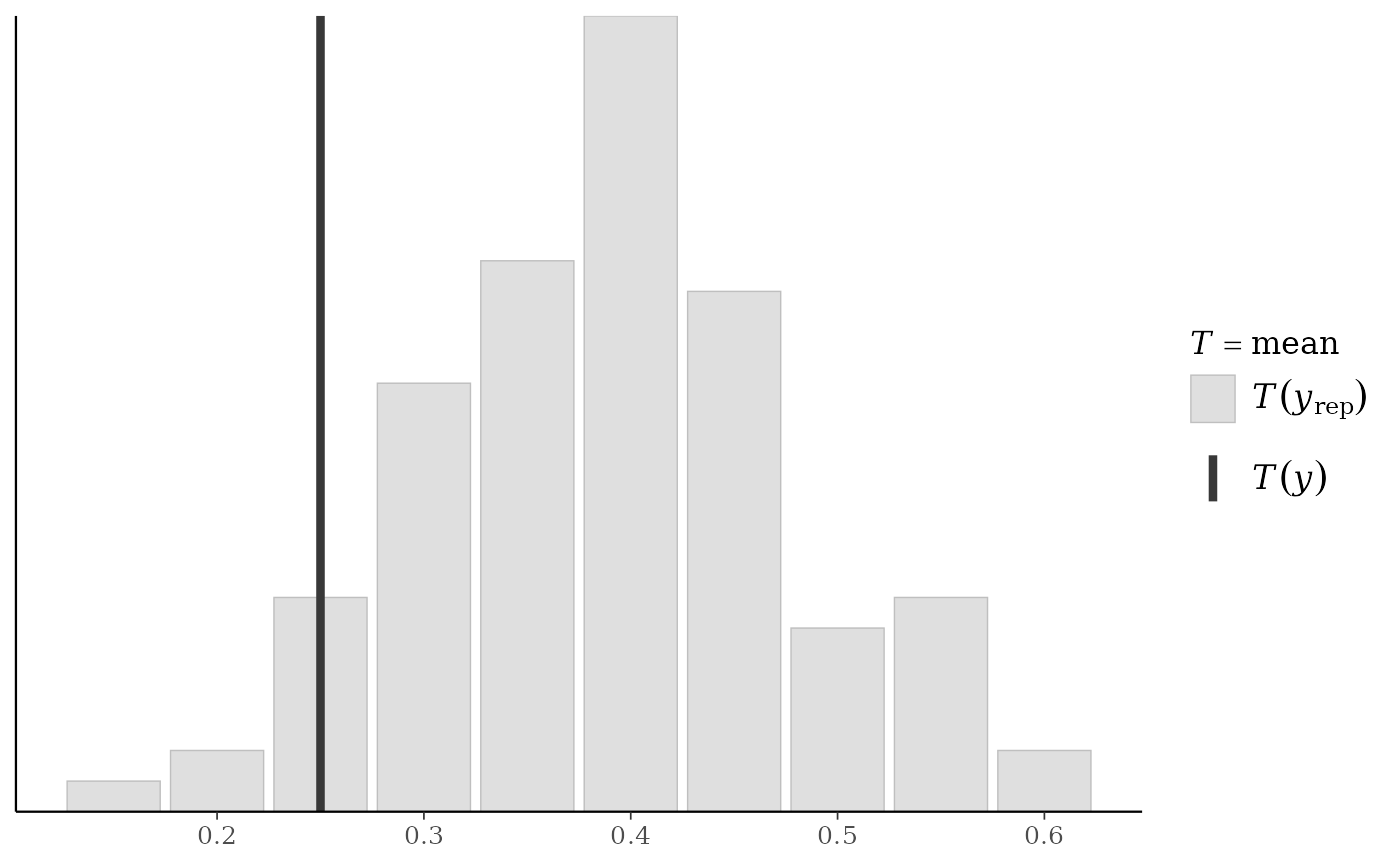# can define the function in the 'stat' argument instead of
# using its name but then the legend doesn't include the function name
ppc_stat(y, yrep, stat = function(y) quantile(y, 0.25))
#> stat_bin() using bins = 30. Pick better value with binwidth.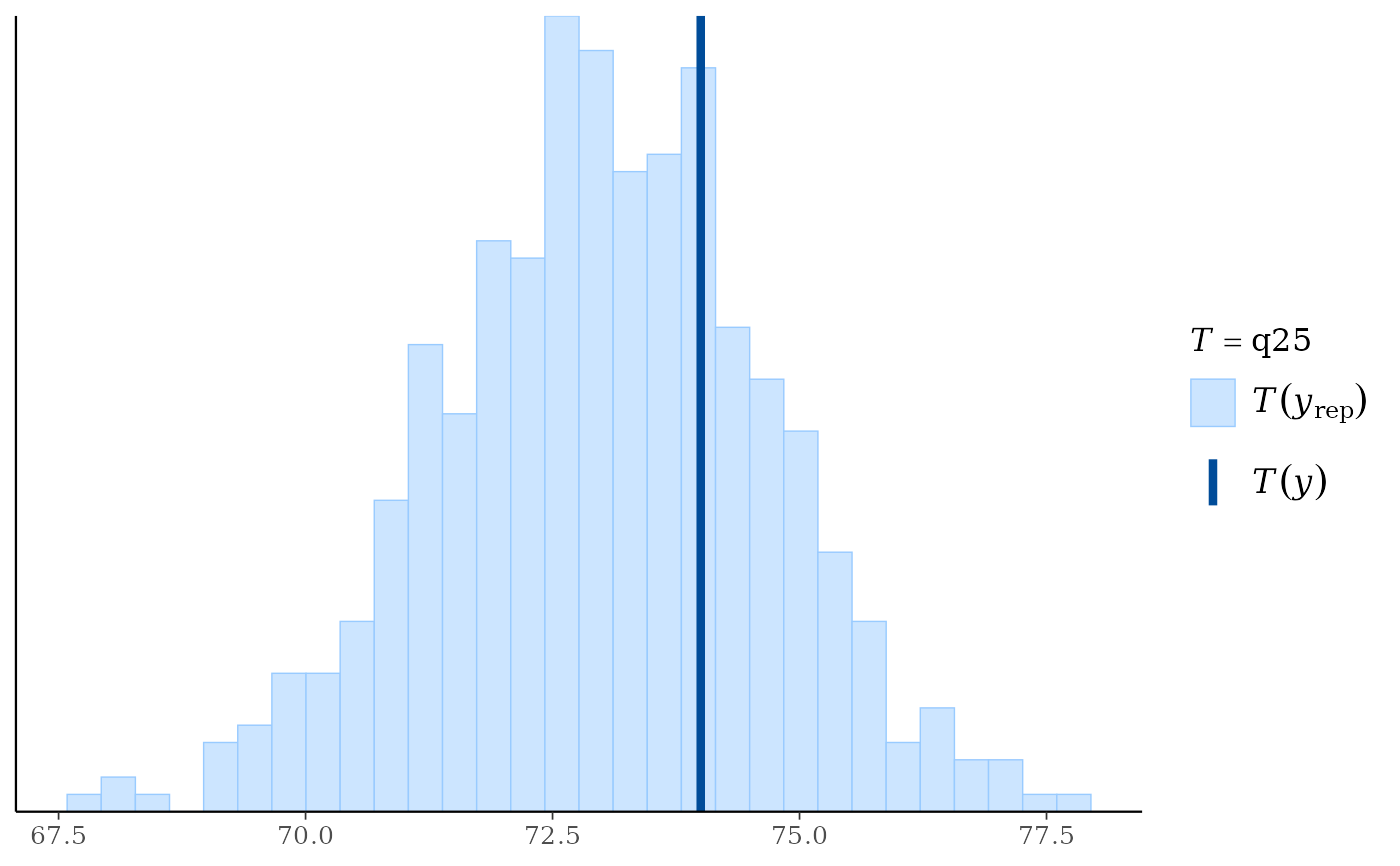# plots by group
color_scheme_set("teal")
group <- example_group_data()
ppc_stat_grouped(y, yrep, group)
#> stat_bin() using bins = 30. Pick better value with binwidth.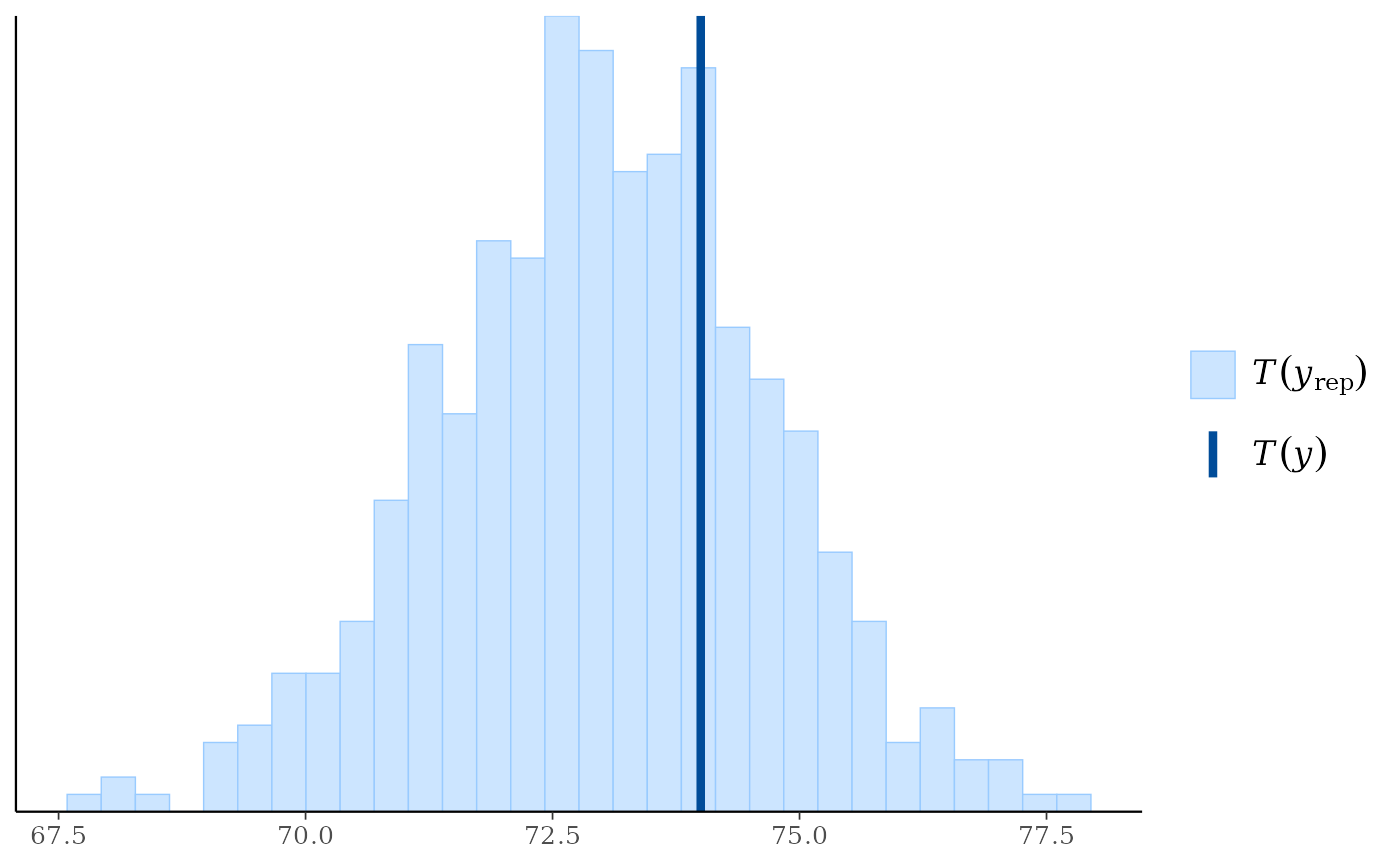ppc_stat_grouped(y, yrep, group) + yaxis_text()
#> stat_bin() using bins = 30. Pick better value with binwidth.# force y-axes to have same scales, allow x axis to vary
ppc_stat_grouped(y, yrep, group, facet_args = list(scales = "free_x")) + yaxis_text()
#> stat_bin() using bins = 30. Pick better value with binwidth.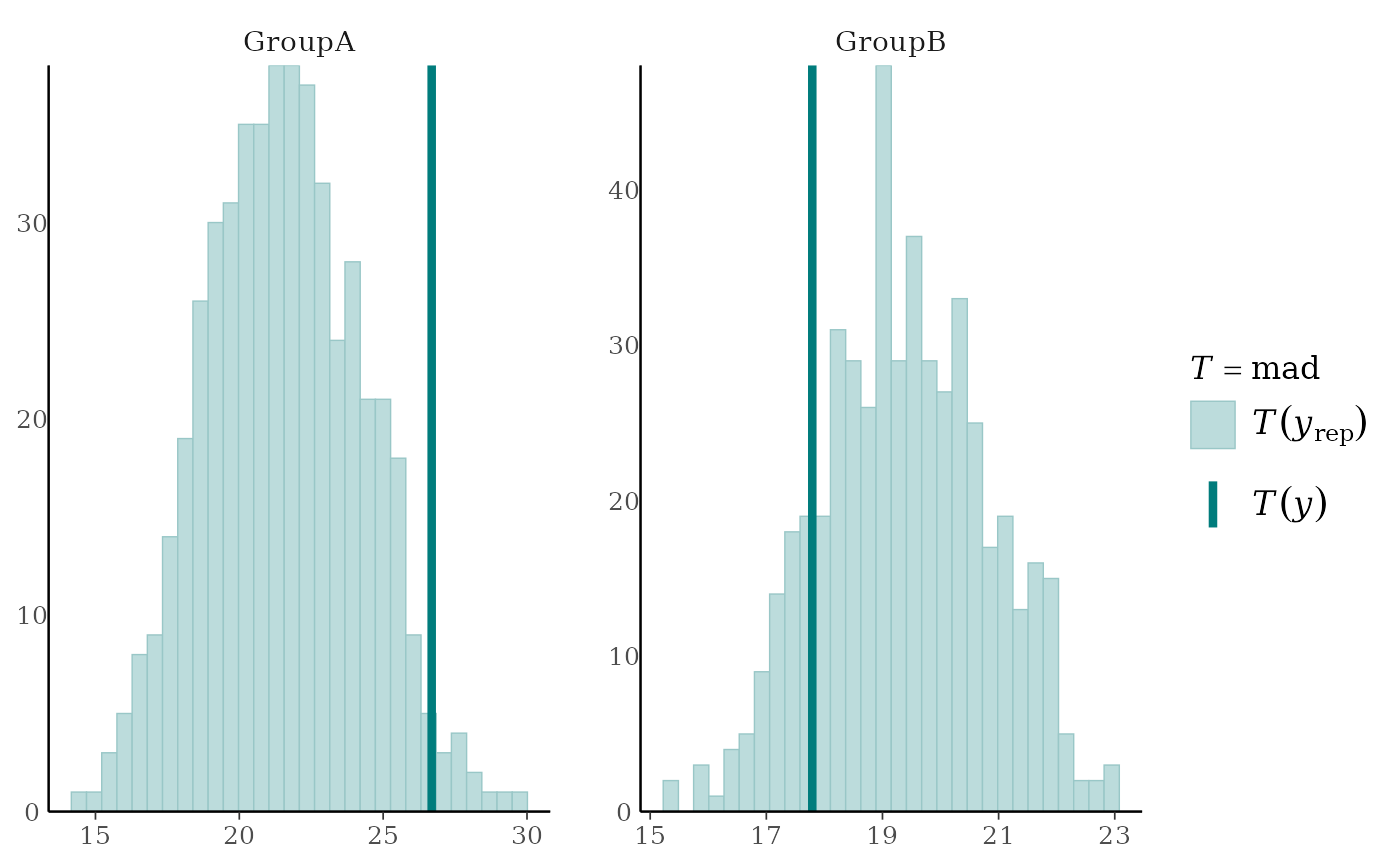# the freqpoly plots use frequency polygons instead of histograms
ppc_stat_freqpoly(y, yrep, stat = "median")
#> stat_bin() using bins = 30. Pick better value with binwidth.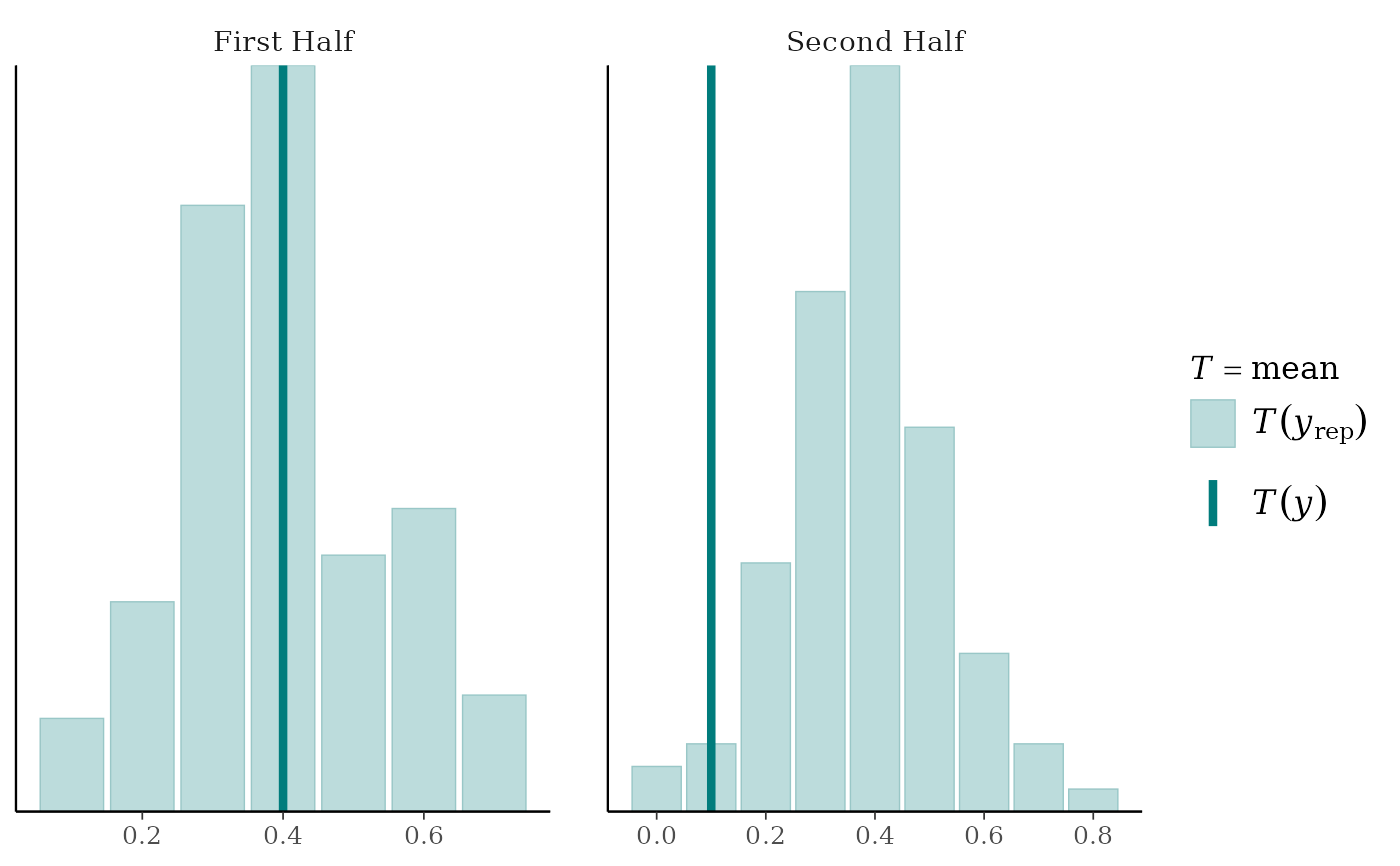ppc_stat_freqpoly_grouped(y, yrep, group, stat = "median", facet_args = list(nrow = 2))
#> stat_bin() using bins = 30. Pick better value with binwidth.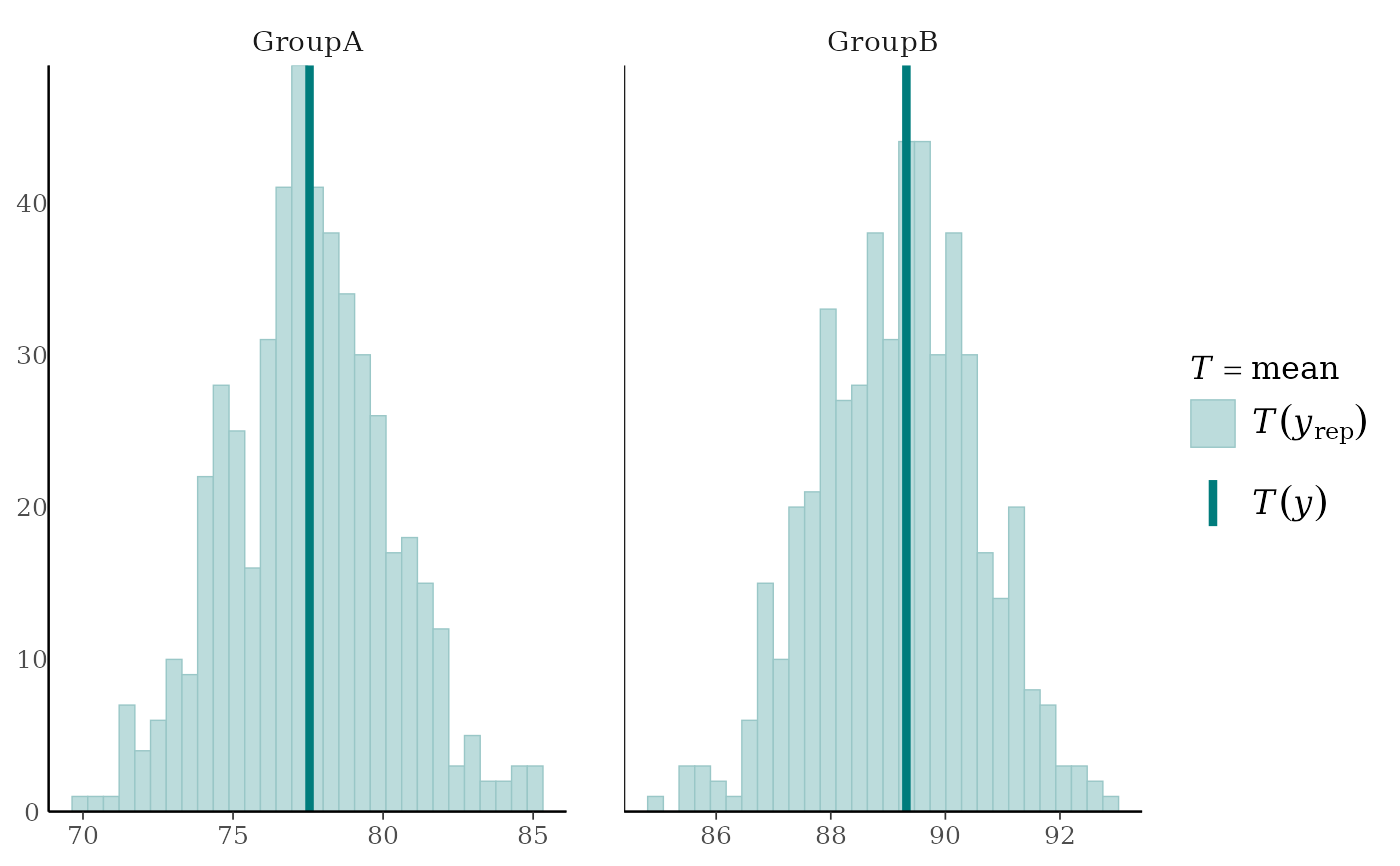# ppc_stat_2d allows 2 statistics and makes a scatterplot
bayesplot_theme_set(ggplot2::theme_linedraw())
color_scheme_set("viridisE")
ppc_stat_2d(y, yrep, stat = c("mean", "sd"))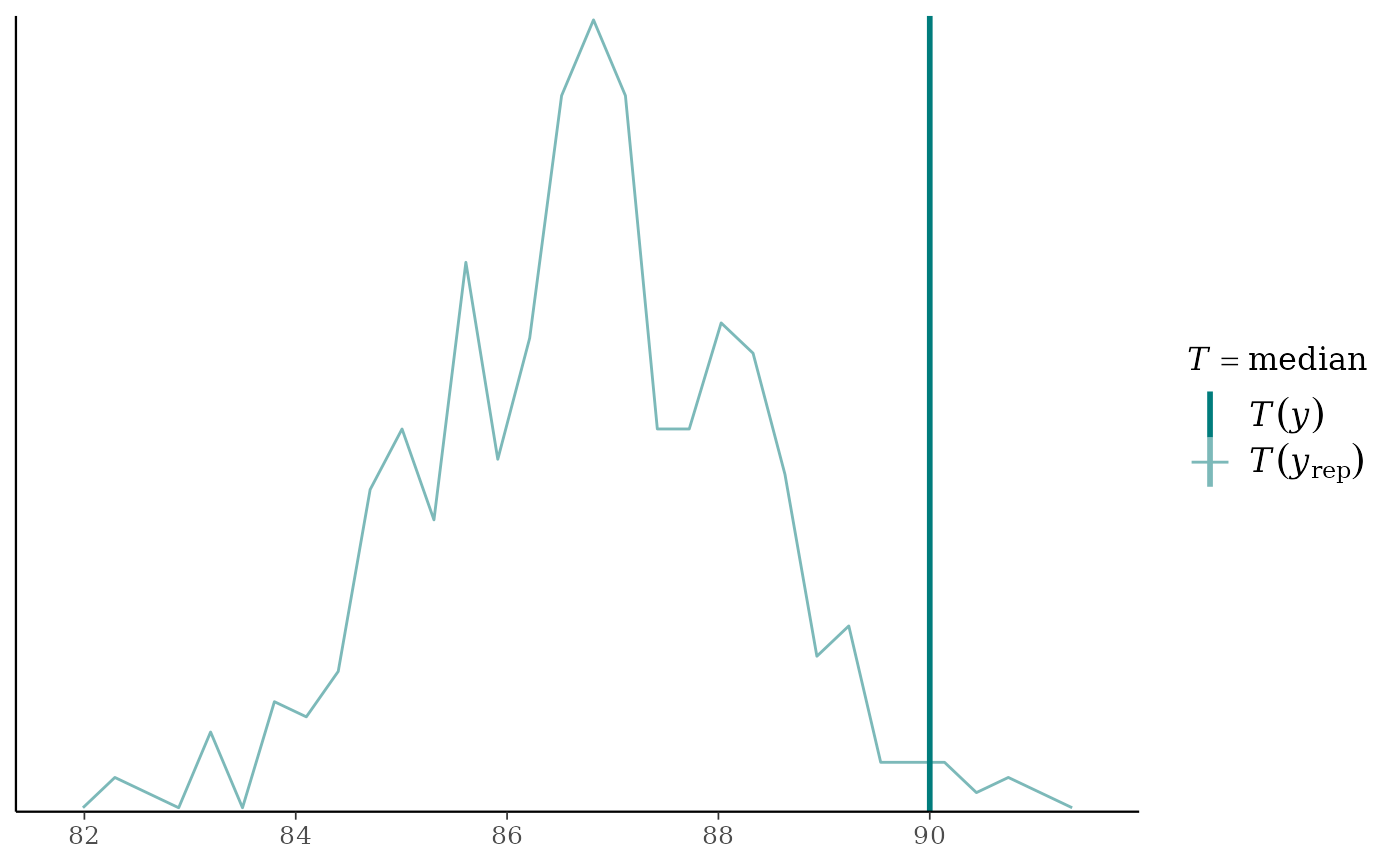bayesplot_theme_set(ggplot2::theme_grey())
color_scheme_set("brewer-Paired")
ppc_stat_2d(y, yrep, stat = c("median", "mad"))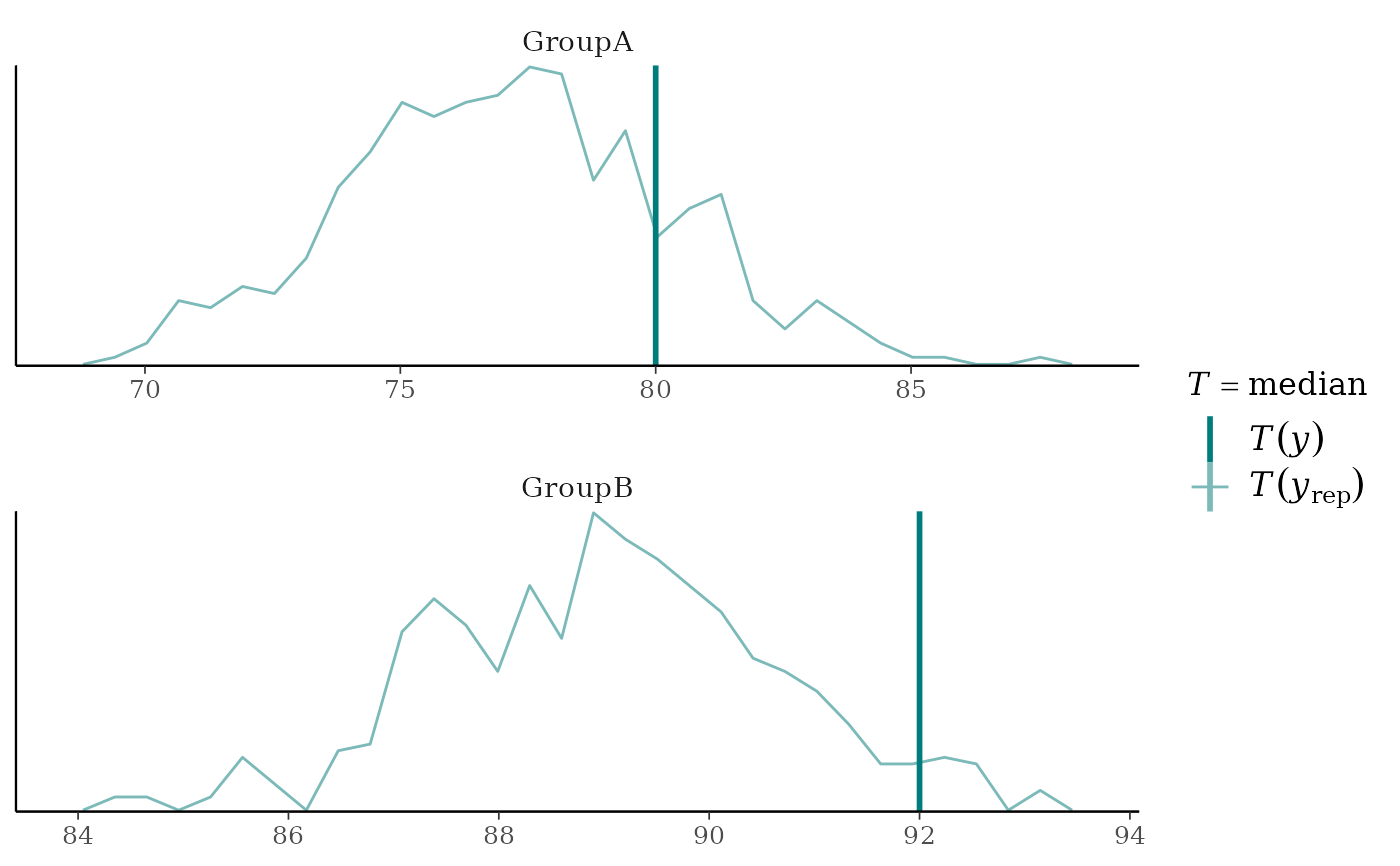# reset aesthetics
color_scheme_set()
bayesplot_theme_set()